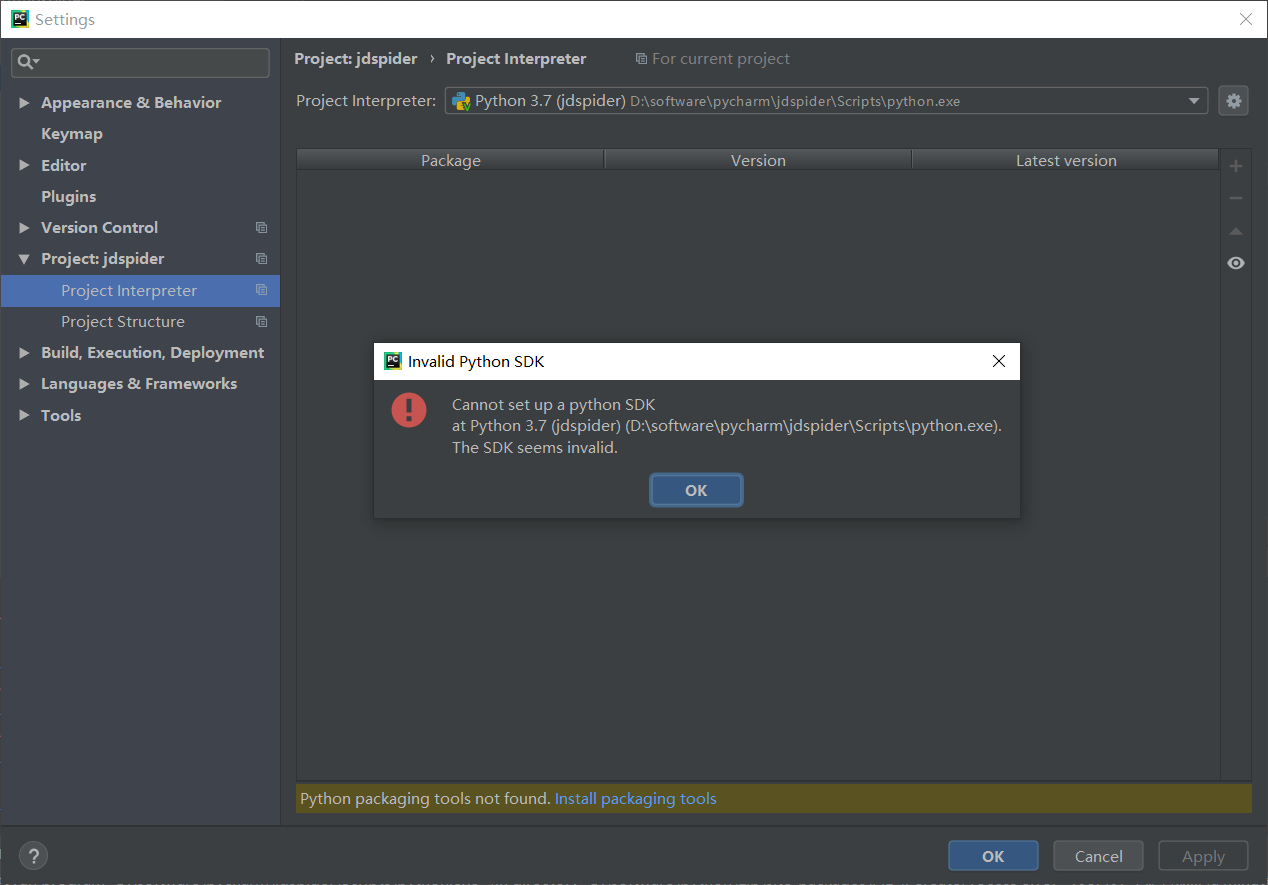pycharm误删script文件，恢复后运行程序出现CreateProcess error=193, %1 不是有效的 Win32 应用程序

pycharm误删了script文件，恢复后再次运行程序显示1个回答shyJump 第一个目录不是pycharm里的目录吗？还需要重新安装python吗
8 个月之前 回复window10版pycharm调试远程代码文件路径（script path）出现乱码

pycharm里运行.sh文件与在shell里运行，有什么不同

#文件random_walk.py from random import choice class Randomwalk(): def _ _init_ _ (self, num_points=500): """初始化随机漫步的属性""" self.num_points = num_points # 所有随机漫步都始于（0,0） self.x_values =  self.y_values =  def fill_walk(self): """计算随机漫步所包含的所有点” """ # 不断漫步， 直到列表达到指定的长度 while len(self.x_values) < self.num_points: # 决定前进方向以及沿着个方向前进的距离 x_direction = choice([1, -1]) x_distance = choice([0, 1, 2, 3, 4]) x_step = x_direction * x_distance y_direction = choice([1, -1]) y_distance = choice([1, 2, 3, 4]) y_step = y_direction * y_distance # 拒绝原地踏步 if x_step == 0 and y_step == 0: continue # 计算下一个点的x值和y值 next_x = self.x_values[-1] + x_step next_y = self.y_values[-1] + y_step self.x_values.append(next_x) self.y_values.append(next_y) #文件rw_visual.py import matplotlib.pyplot as plt from random_walk import Randomwalk rw = Randomwalk() rw.fill_walk() plt.scatter(rw.x_values, rw.y_values, s=15) plt.show() 在idle里面能出结果，在pycharm中没报错，但没结果

#文件 random_walk.py from random import choice class RandomWalk(): """生成一个随机漫步数据的类""" def __init__(self, num_points=500): """初始化随机漫步的属性""" self.num_points = num_points #所有随机漫步都始于（0,0） self.x_values =  self.y_values =  def fill_walk(self): """计算随机漫步所包含的所有点” """ #不断漫步， 直到列表达到指定的长度 while len(self.x_values) < self.num_points: #决定前进方向以及沿着个方向前进的距离 x_direction = choice([1,-1]) x_distance = choice([0,1,2,3,4]) x_step = x_direction * x_distance y_direction = choice([1,-1]) y_distance = choice([1,2,3,4]) y_step = y_direction * y_distance #拒绝原地踏步 if x_step == 0 and y_step == 0: continue #计算下一个点的x值和y值 next_x = self.x_values[-1] + x_step next_y = self.y_values[-1] + y_step self.x_values.append(next_x) self.y_values.append(next_y) #文件rw_visual.py import matplotlib.pyplot as plt from random_walk import RandomWalk #创建一个RandomWalk实例， 并将其包含的点都绘制出来 rw = RandomWalk() rw.fill_walk() plt.scatter(rw.x_values,rw.y_values, s=15) plt.show() 在pycharm里运行rw_visual.py，半天没有反应，是代码的问题还是计算机的问题，码放高手指点一下，谢谢！

Windows系统中，pycharm怎么运行.sh文件？

pycharm执行图形程序不出现图形界面

pycharm执行图形程序不出现图形界面，只出现Process finished with exit code 0，之前还是能 显示出图形的，为什么突然不好用了？

windows下用pycharm读取txt文件编码问题

pycharm2019，每次打开上一次运行过的文件，就会抱错如图所示，求问得如何解决

Pycharm写完程序后如何生成类似于VS中.exe的文件

Python脚本运行问题，在pycharm中运行会出错

pycharm中matplotlib运行出现问题

Pycharm 无法打包生成exe文件

pycharm中如何用tensoflow-gpu运行文件

pycharm创建的文件都是GBK的编码格式，如何修改pycharm的设置，让创建的文件默认为utf-8

“亚马逊丛林里的蝴蝶扇动几下翅膀就可能引起两周后美国德州的一次飓风……” 这句人人皆知的话最初用来描述非线性系统中微小参数的变化所引起的系统极大变化。 而在更长的时间尺度内，我们所生活的这个世界就是这样一个异常复杂的非线性系统…… 水泥、穹顶、透视——关于时间与技艺的蝴蝶效应 公元前3000年，古埃及人将尼罗河中挖出的泥浆与纳特龙盐湖中的矿物盐混合，再掺入煅烧石灰石制成的石灰，由此得来了人...

loonggg读完需要3分钟速读仅需 1 分钟大家好，我是你们的校长。我之前讲过，这年头，只要肯动脑，肯行动，程序员凭借自己的技术，赚钱的方式还是有很多种的。仅仅靠在公司出卖自己的劳动时...

MySQL数据库面试题（2020最新版）

HashMap底层实现原理，红黑树，B+树，B树的结构原理 Spring的AOP和IOC是什么？它们常见的使用场景有哪些？Spring事务，事务的属性，传播行为，数据库隔离级别 Spring和SpringMVC，MyBatis以及SpringBoot的注解分别有哪些？SpringMVC的工作原理，SpringBoot框架的优点，MyBatis框架的优点 SpringCould组件有哪些，他们...

《经典算法案例》01-08：如何使用质数设计扫雷（Minesweeper）游戏

《Oracle Java SE编程自学与面试指南》最佳学习路线图（2020最新版）